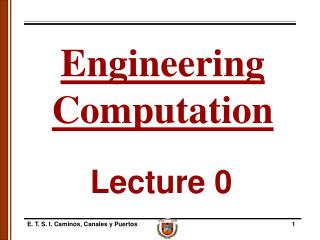DownloadDownload PresentationLecture 0

# Lecture 0

Download Presentation## Lecture 0

- - - - - - - - - - - - - - - - - - - - - - - - - - - E N D - - - - - - - - - - - - - - - - - - - - - - - - - - -
##### Presentation Transcript

1. EngineeringComputation Lecture 0 E. T. S. I. Caminos, Canales y Puertos

2. Introduction • Objectives • 1. Introduction (quite ambitious!) to numerical methods for engineering as a general and fundamental tool for all engineering disciplines. We plan to cover (almost) the main topics of numerical analysis and basic concepts of probability & statistics. • 2. Programming will be essential; we will use commercial software widely used in science and engineering: MATLAB and Excel. • 3. We will illustrate and discuss how numerical methods are used in practice. We will consider examples from Engineering. E. T. S. I. Caminos, Canales y Puertos

3. Introduction • ENGCOMP Course overview • 1. Approximation, errors, and precision. • 2. Finding roots of functions of one variable. • 3. Simultaneous linear equations: • - tri-diagonal, LU decomposition, iterative solutions; • - norms, condition number, operation counting. • 4. Numerical methods for ODE’s. • 5. Introduction to numerical solutions of PDE’s. • 6. Introductory probability & statistics. • 7. Curve-fitting, regression, and cubic splines. • 8. Numerical integration and differentiation. E. T. S. I. Caminos, Canales y Puertos

4. Introduction • Why are Numerical Methods so widely used in Engineering? • Engineers use mathematical modeling (equations and data) to describe and predict the behavior of systems. • Closed-form (analytical) solutions are only possible and complete for simple problems (geometry, properties, etc.). • Computers are widely available, powerful, and (relatively) cheap. • Powerful software packages are available (special or general purpose). E. T. S. I. Caminos, Canales y Puertos

5. Introduction • A few applications of Numerical Methods in Engineering: • Structural/mechanical analysis, design, and behavior. • Communication/power • Network simulation • Train and traffic networks • Computational Fluid Dynamics (CFD): • Weather prediction • Groundwater & pollutant movement E. T. S. I. Caminos, Canales y Puertos

6. Introduction • Why study Numerical Methods? • Numerical Analysis is a Discipline: • Need to understand concepts and theory • - Know what problems can be solved. • - Know what problems cannot be solved, or when problems will be troublesome. • Need to understand methods and techniques • - Know why methods work, or judge when they are working. • - Be able to create or modify tools (software) as needed. • - Evaluate errors, convergence, and stability of arithmetic approximations. E. T. S. I. Caminos, Canales y Puertos

7. Introduction • Why study Numerical Methods? (continued) • Use of Numerical Methods is an Art: • Numerical methods are approximate. • The most appropriate method(s) is not always obvious. • Evaluating precision and accuracy is an essential part of the process. E. T. S. I. Caminos, Canales y Puertos

8. Introduction Instructors:Prof. Amparo Gil Departamento de Matemática Aplicada y CC. De la Computación Escuela de Ingenieros de Caminos Universidad de Cantabria e-mail: amparo.gil@unican.es Office Hours: by appointment via e-mail. Visiting Prof. Sarah Hallerberg Departamento de Matemática Aplicada y CC. De la Computación Escuela de Ingenieros de Caminos Universidad de Cantabria E. T. S. I. Caminos, Canales y Puertos

9. Introduction • Course computing framework: • Spreadsheets • – Microsoft Excel. • Numerical Languages • – MATLAB • Electronic Communication by e-mail: • Computer assignments will be submitted as attachments via e-mail: amparo.gil@unican.es • Text files, Excel & MATLAB documents as attachments. • documents will be distributed via the ENGCOMP web page. http://personales.unican.es/gila/UC-Cornell E. T. S. I. Caminos, Canales y Puertos

10. Introduction • ENGCOMP Course Materials • Required Textbook and Notes: • Chapra & Canale, Numerical Methods for Engineers, 4th Ed., 2002 • Computer sessions (recommended texts): • Palm, Introduction to MATLAB for Engineers. • The MathWorks, The Student Edition of MATLAB. • Pratap, Getting Started with MATLAB. • Additional material will be available at the course website, e.g.: • "Introduction to Spreadsheets: Using Microsoft EXCEL" • "Primer on MATLAB" E. T. S. I. Caminos, Canales y Puertos

11. Selected bibliography • Datta, NB, “Numerical linear algebra and applications”, 1995, Pacific Grove, California: Brooks-Cole, cop. • Gil, A., Segura, J., Temme, NM, “Numerical Methods for Special Functions”, 2007, SIAM. • Kincaid D., Cheney W., “Análisis Numérico”. Addison-Wesley Iberoamericana,1994. • Lambert, J.D., “Numerical Methods for Ordinary Differential Equations”, 1973, John Wiley & Sons. • Mitchell, A.R., Griffiths, D.F., “The Finite Difference Method in Partial Differential Equations”, 1980, Wiley, London. • Schwartz, H.R., “Finite Element Methods”, 1998, Academic Press, London. E. T. S. I. Caminos, Canales y Puertos

12. Introduction • Weekly Assignments • Problem Sets (PS) • - teams of 3; work together, check each other • - teams to be formed at the end of September. • Computer Assignments (CA) due Friday • teams of 3; work together, learn from each other • submit electronically • Assignment submissions must follows the standards described on the course web page. E. T. S. I. Caminos, Canales y Puertos

13. Introduction • Schedule: • Monday, Wednesday: 8:30-10:30 (2 sessions of 50’ each) Lectures • Friday: 8:30-9:30 Computer Session (at the computer lab of the department of Applied Math. and Comput. Science). E. T. S. I. Caminos, Canales y Puertos

14. Contributions to the final grade: • Computer Assignments (CA) 20% • For 2 Prelims 40% • Final Exam 20% • Attendance & participation & various 20% E. T. S. I. Caminos, Canales y Puertos

15. Preliminary exams: • Prelim 1: To be announced (most probably at the end of October). • Prelim 2: To be announced. • Final exam • To be announced E. T. S. I. Caminos, Canales y Puertos

16. … and that’s all for today! E. T. S. I. Caminos, Canales y Puertos